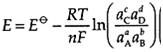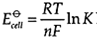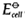## Electrochemistry and ion concentration, Chemistry

Assignment Help:

ELECTROCHEMISTRY AND ION CONCENTRATION

Activity dependence of cell voltage:

Measurement of galvanic cell potentials away from the standard state provides a convenient method for measuring ion activity and activity coefficient. As ion concentration is varied in a half-cell to vary ion activity. Equilibrium position of the half cell reduction reaction changes and that causes a change in the half-cell potential. The relationship between the activity and half-cell potential is given by the Nernst equation:for the general half-cell reduction reaction:

aA+bB+ne-→cC+dD

Cells at equilibrium:

When a cell is at equilibrium, Ecell=0 (the 'flat battery' condition) and Q=K, the equilibrium constant for the reaction (see Topic C1). This gives the expression: which allows calculation of equilibrium constants (see Topic C1) for cell reactions from calculated or measured values.which allows calculation of equilibrium constants for cell reactions from calculated or measuredvalues.

#### Cu2+ will have the following electronic configuration, Cu 2+ will have the...

Cu 2+ will have the following electronic configuration: (1) 1s 2 , 2s 2 , 2p 6 , 3s 2 , 3p 6 , 3d 10 , (2) 1s 2 , 2s 2 , 2p 6 , 3s 2 , 3p 9 , 4s 1     (3) 1s 2 , 2s 2 ,

#### The wave nature of an electron was first given, The wave nature of an elect...

The wave nature of an electron was first given by: (1) De-Broglie (2)Heisenberg (3)Mosley (4)Sommerfield Ans:  De-Broglie

#### Structure of 2-ethylbutanoic acid, 2-Ethylbutanoic acid can be prepared by ...

2-Ethylbutanoic acid can be prepared by a malonic ester synthesis

examples

#### #micrcosmic salts, #why it is caled so ..

#why it is caled so ..

#### Kinetics and equilibrium, 2NO(g)+2H2O yeilds N2+2H2O Write the rate law for...

2NO(g)+2H2O yeilds N2+2H2O Write the rate law for the pervious reaction if doubling the concentration of H2 doubles the reaction rate, and if doubling the concentration of NO incre

#### Choloroplatinic acid metod, A Chloroplatinic of a diacid base contain 39% o...

A Chloroplatinic of a diacid base contain 39% of platinum what is the molecular weight of the base

#### Thermodynamicsppp, Ask question #Minimum 100 words acceptep

Ask question #Minimum 100 words acceptep

#### Preparation of ethane, Ask question #Minimum 25 words accepted#

Ask question #Minimum 25 words accepted#